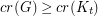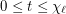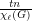# Albertson, Michael O.

## Crossing numbers and coloring ★★★

Author(s): Albertson

We letdenote the crossing number of a graph.

Conjecture   Every graphwithsatisfies.

Keywords: coloring; complete graph; crossing number

## Partial List Coloring ★★★

Author(s): Albertson; Grossman; Haas

Conjecture   Letbe a simple graph withvertices and list chromatic number. Suppose thatand each vertex ofis assigned a list ofcolors. Then at leastvertices ofcan be colored from these lists.

Keywords: list assignment; list coloring